出租广告位,需要合作请联系站长

+关注

2021-02(16)

2021-03(41)

3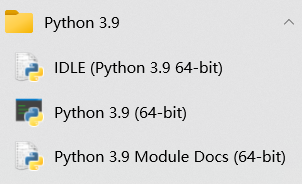1. IDLE自带简单开发环境
2. 交互式命令行程序
3. manuals官方技术文档
4. module已安装模块文档

5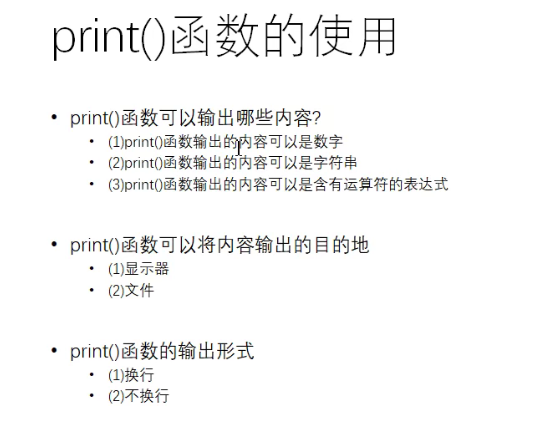1. print(520)
2. print('helloworld')
3. print(3+2)
4. #将数据输出到文件中,1.路径存在2.使用file=
5. fp=open('D:/text.txt','a+')#a+：如果文件不存在就创建，存在就在文件内容的后面继续追加
6. print('helloworld',file=fp)
7. fp.close()
8. #不进行换行输出（输出内容在一行当中）
9. print('hello','world','pyhton')

6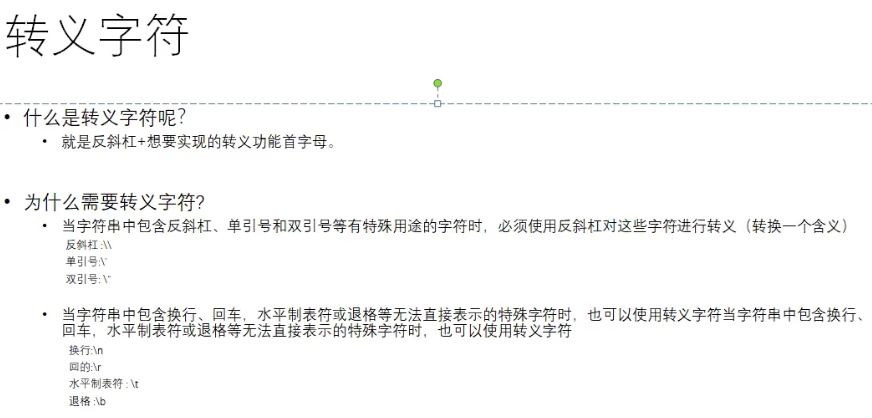1. print('hello\nworld')
2. print('hello\tworld')#四个字符一组
3. print('hello000\tworld')
4. print('hello\rworld\rfhd')#进行覆盖
5. print('hello\bworld')#退格，o没了
6. print('http:\\\www.com')
7. print('老师说:\'大家好\'')
8. #原字符，不希望字符串中的转义字符起作用，在字符串前加上r或者R
9. #注意事项，最后一个字符不能是反斜杠
10. print(r'hello\nworld')

8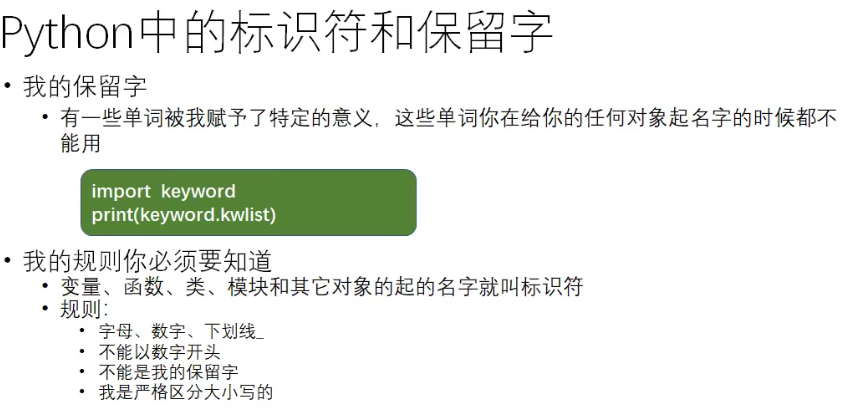1. import keyword
2. print(keyword.kwlist)#查看保留字

9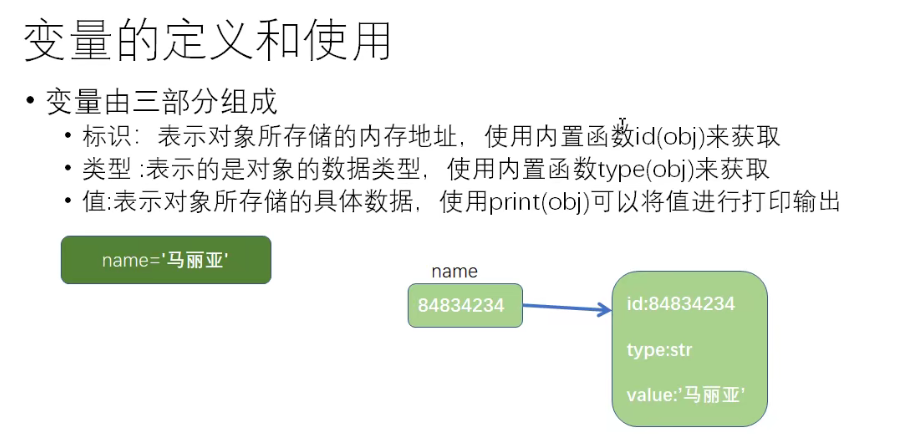13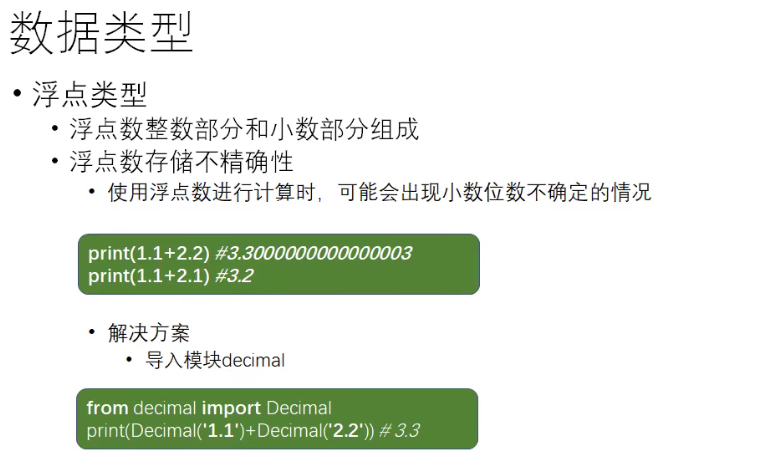15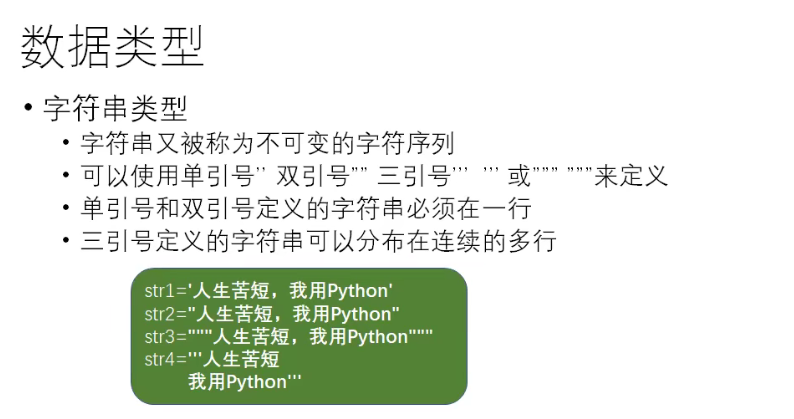19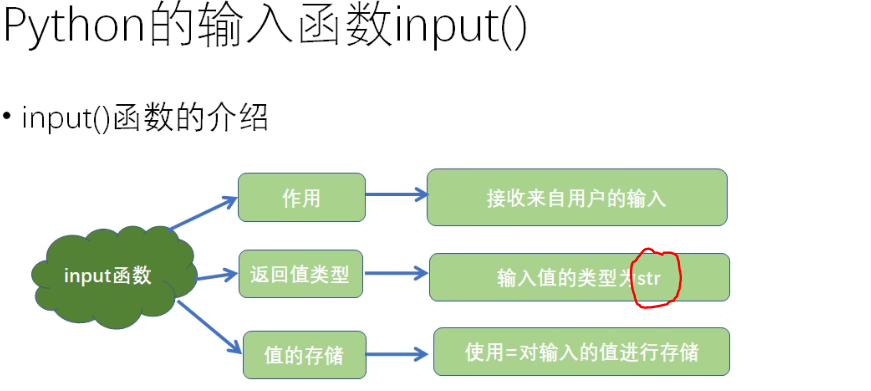21

算数运算符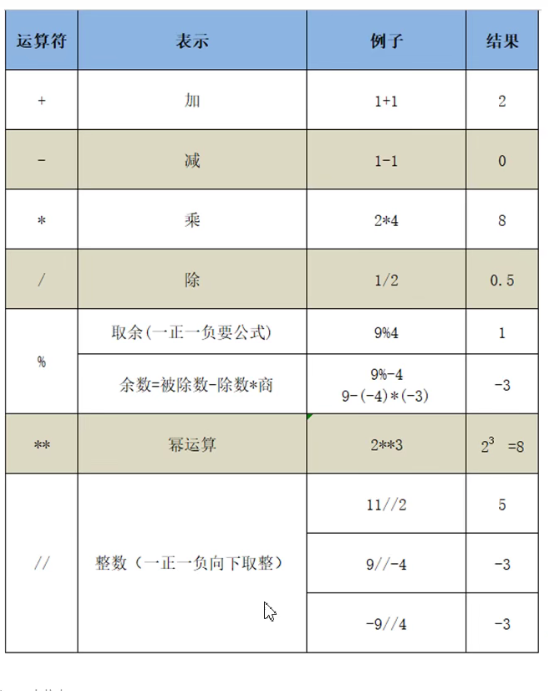1. print(1/3)#除法运算0.3333333333333333
2. print(1//3)#整除运算0
3. print(2**2)#幂运算符：2的2次方
4. print(2**3)#2的3次方
5. print(9%-4)#-3

22

1. #支持解包赋值
2. a,b,c=20,30,40
3. print(a,b,c)
4. a=b=c=10#链式赋值，abc的id一样
5. print(a,id(a))#10 1627767990864
6. print(b,id(b)#10 1627767990864
7. print(c,id(c))#10 1627767990864

23

== 比较的是值

is 比较的是对象的标识

1. a=10
2. b=10
3. print(a==b)#true
4. print(a is b)#true

24

1. s='helloworld'
2. print('w' in s)#true
3. print('k' in s)#false
4. print('o' not in s)#false
5. print('p' not in s )#true

25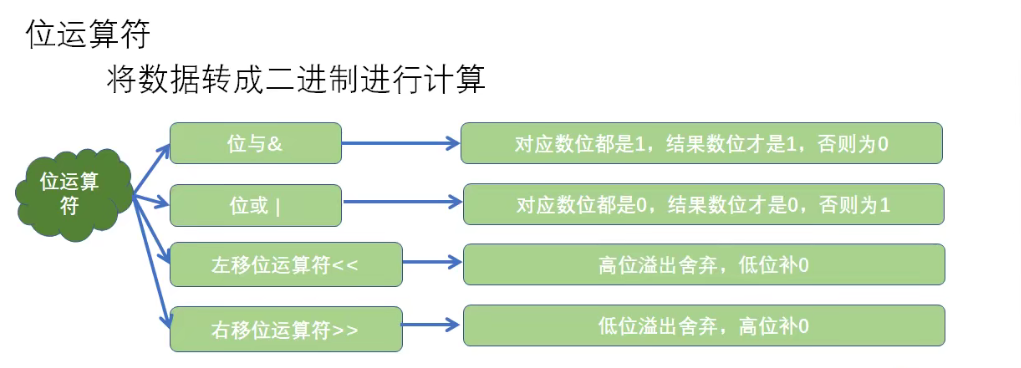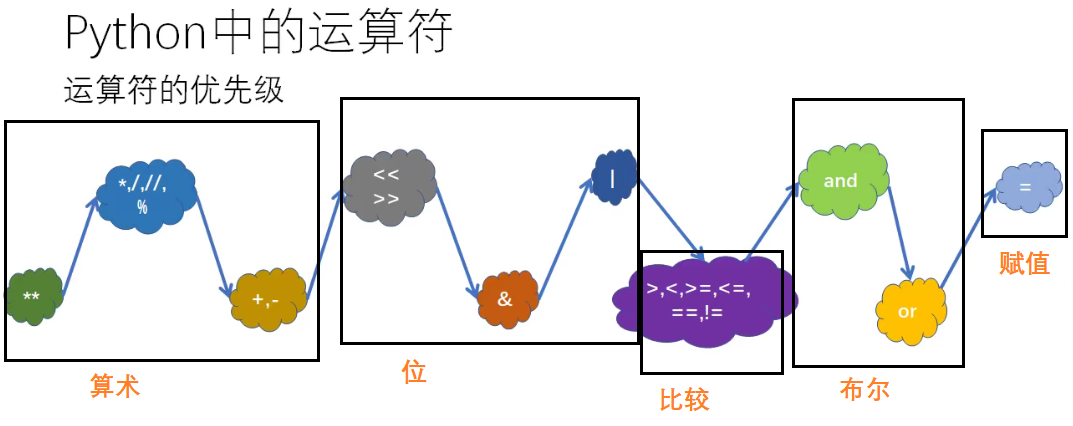28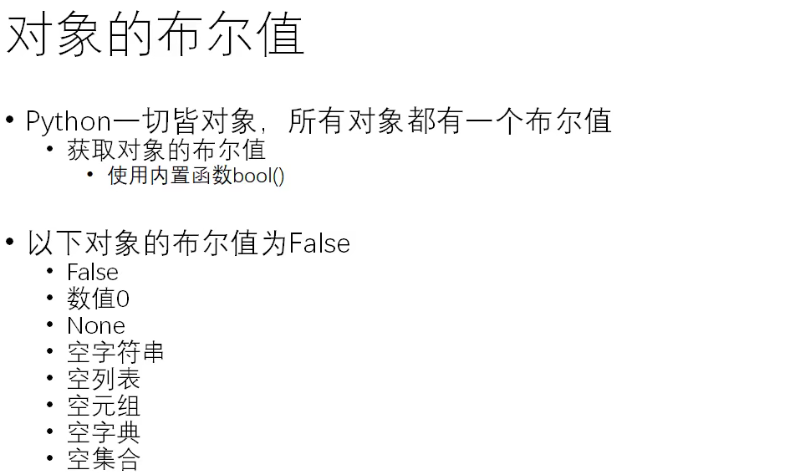1. #以下对象的布尔值均是False
2. print(bool(False))
3. print(bool(0))
4. print(bool(0.0))
5. print(bool(None))
6. print(bool(''))#空字符串
7. print(bool(""))
8. print(bool([]))#空列表
9. print(bool(list()))
10. print(bool(()))#空元祖
11. print(bool(tuple()))
12. print(bool({}))#空字典
13. print(bool(dict()))
14. print(bool(set()))#空集合

31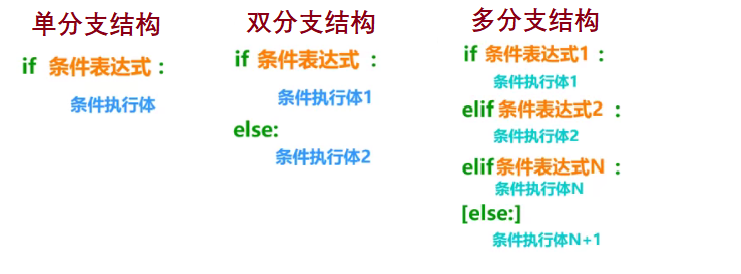32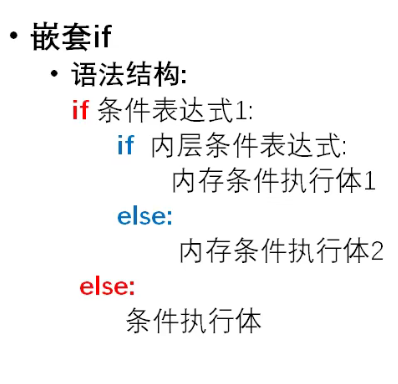33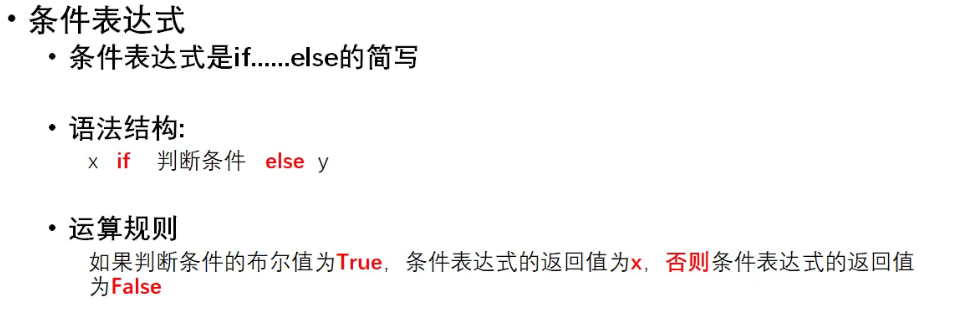34

PASS语句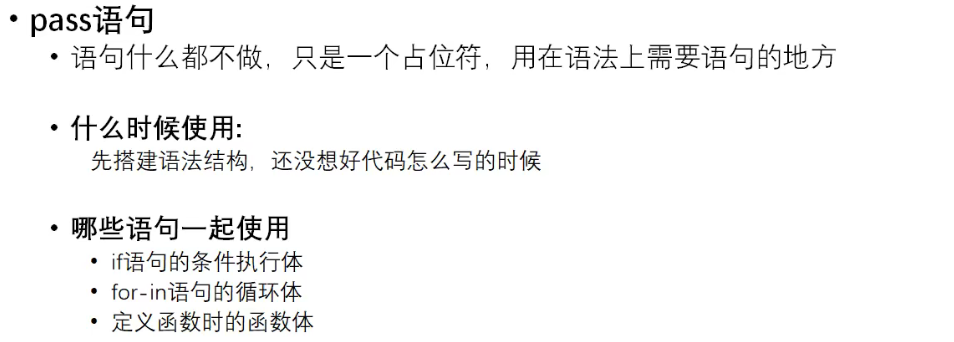8 0

pdf(new) 更多>